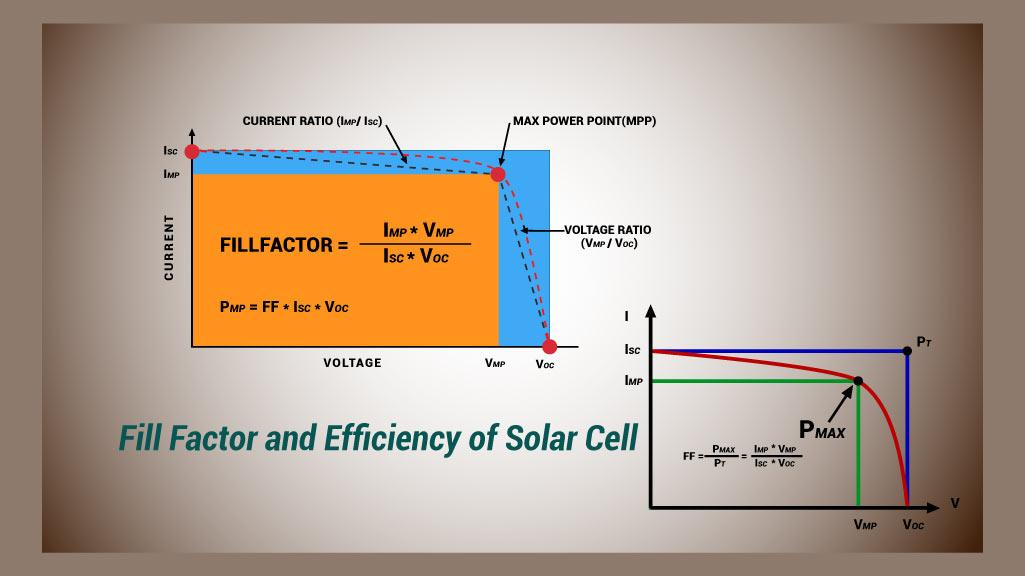# Fill factor

## illustrates the concept of fill factor

can be seen from the figure, short-circuit photocurrent is I-V curve intercept on the ordinate axis, and the open-circuit photovoltage curve was cross- coordinate axis intercept. Short-circuit current is the maximum current of the battery can be generated, corresponding to the load resistance is zero, then the output voltage of the battery is zero. A battery open-circuit voltage is the maximum voltage that can be generated, corresponding to the load resistance is infinite, then the output current of the battery is zero. Label points (x) on the curve corresponding to the current and voltage of the current and voltage at the maximum output power, the points corresponding to the rectangular area is the maximum output power. The point (this is not actually) corresponding to a rectangular area having a short-circuit current and open circuit voltage of the battery is the maximum power that can be produced theoretically. Area (maximum output power of the battery can)

marked points corresponding to the maximum area (theoretical power) ratio is the fill factor. Energy conversion efficiency

Obviously, the fill factor is an important parameter influence cell output performance of certain open circuit voltage and short circuit current, conversion efficiency of the battery depends on the fill factor, large fill factor is high . Short-circuit current and open circuit voltage of the battery are the two most important parameters, the higher short-circuit current and open-circuit voltage is generated based on higher energy conversion efficiency.fill factor (FF) is the corresponding rectangular area (ideal shape) comparing a measure of the maximum power of the area of ​​the open short circuit the solar cell I-V characteristic curves contained. Clearly, the FF should be close to 1 (i.e. 100%) as much as possible, but the p-n junction characteristics of an exponential function of 1 will prevent it. The larger the fill factor, the higher the quality of the solar cell. Typical values ​​of the FF is generally 60 to 85%, determined by the device structure and materials of the solar cell.

## factors

fill factor is the ratio of the maximum power of the solar cell and the open circuit voltage and short circuit current of the product, is an important parameter for evaluating the output characteristics of the solar cell. The higher its value, indicates that the output characteristics of the solar cell close to the rectangle, the higher photoelectric conversion efficiency. Factors

Effect fill factor are: the short-circuit current; open current; series resistance; parallel resistance; temperature; spectral intensity.

fill factor mainly depends on the material properties of the solar cell itself, dependent on the light source employed is not strong.

studies have confirmed the impact of the solar cell output characteristic of internal factors in series, parallel resistance influence on the fill factor of the maximum: the greater the resistance in series, the smaller parallel resistance, the fill factor will be smaller.

### Irradiance influence on the fill factor

affect the solar cell output characteristic of most external factors than the sunlight intensity days. Fill factor changes with the intensity of sunlight has not been clearly expressed. Also, in engineering practice, it has been noted in sunlight the solar cell output characteristics: short-circuit current and the maximum power point current is proportional with the intensity of sunlight, the open-circuit voltage and the maximum power point voltage with the natural logarithm of the intensity of sunshine proportional.

Effect

### wavelength of incident light load and fill factor

fill factor is a graph of the wavelength of incident light and

. With the wavelength of incident light is increased, the fill factor increases and then decreases, and then increases again. Since the spectral response within the range of 365 nm incident light is not a silicon solar cell, the incident light wavelength of 365 nm, the fill factor is small. A solar cell surface is coated with a silicon nitride film blue reflection wavelength of 436 nm of blue light results in a strong incident light is 436 nm smaller the fill factor of the solar cell.

on the whole, with the wavelength of incident light increases, a gradual increase in the photon energy efficiency, fill factor also increases.

Related Articles